# Tree examples for assignment 5

This page has some examples of trees built using the IBranch and ILeaf constructors specified in assignment 5.

{-# LANGUAGE FlexibleContexts #-}
module A05Trees where
import A05Sol hiding (main)
import System.IO

We’ll use this shortcut for a “terminal branch” – one whose left and right children are both empty:

iterm :: a -> ITree a
iterm x = IBranch x ILeaf ILeaf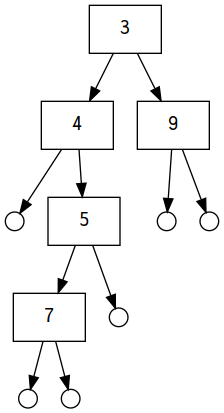t1 :: ITree Int
t1 =
IBranch 3
(IBranch 4
ILeaf
(IBranch 5 (iterm 7) ILeaf))
(iterm 9)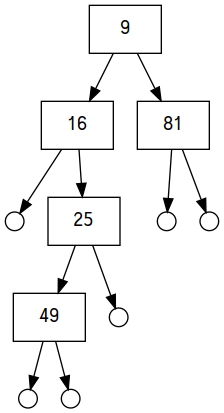The result of fmap square t1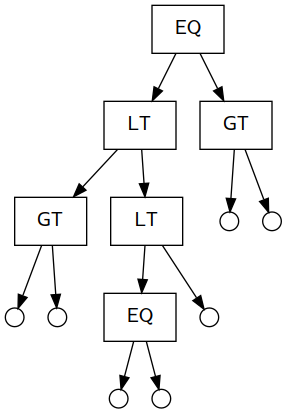t2 :: ITree Ordering
t2 =
IBranch EQ
(IBranch LT
(iterm GT)
(IBranch LT (iterm EQ) ILeaf))
(iterm GT)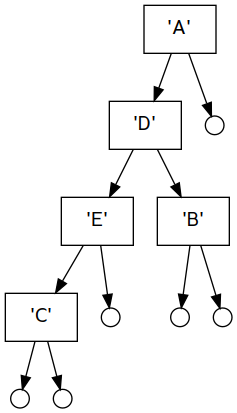t3 :: ITree Char
t3 =
IBranch 'A'
(IBranch 'D'
(IBranch 'E' (iterm 'C') ILeaf)
(iterm 'B'))
ILeaf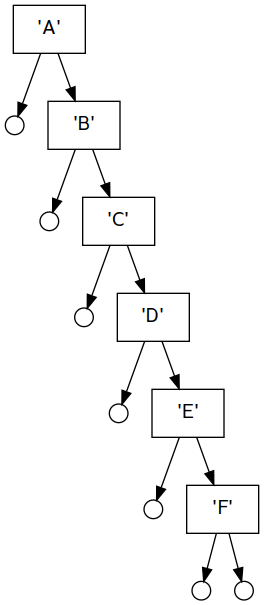alpha :: String
alpha = "ABCDEF"
t4 :: ITree Char
t4 = foldr (flip IBranch ILeaf) ILeaf alpha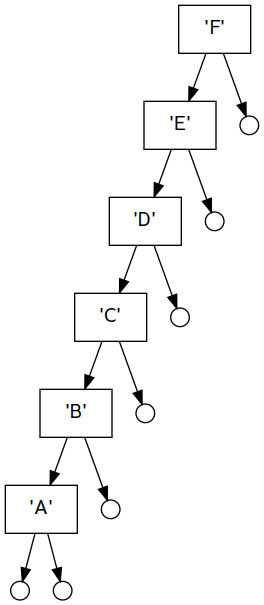t5 :: ITree Char
t5 = foldl (\t x -> IBranch x t ILeaf) ILeaf alpha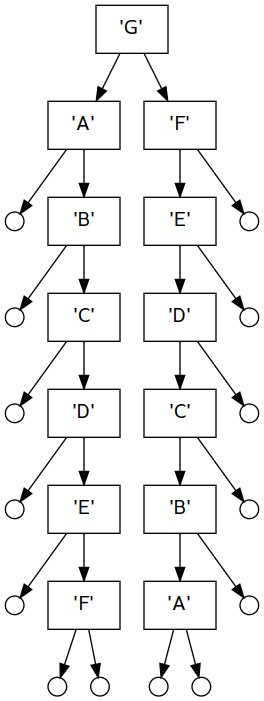t6 :: ITree Char
t6 = IBranch 'G' t4 t5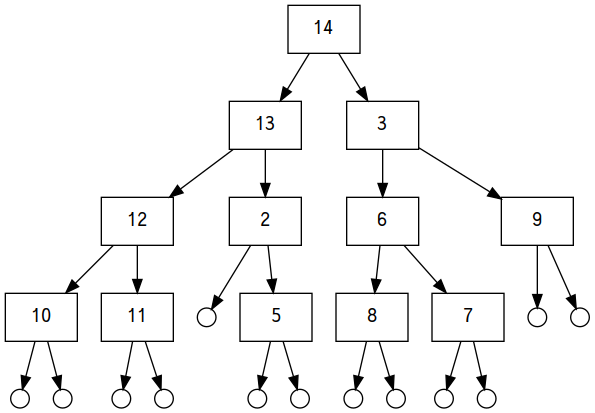t7 :: ITree Int
t7 =
IBranch 14
(IBranch 13
(IBranch 12
(iterm 10)
(iterm 11))
(IBranch 2
ILeaf
(iterm 5)))
(IBranch 3
(IBranch 6
(iterm 8)
(iterm 7))
(iterm 9))

range :: [Int]
range = [21..31]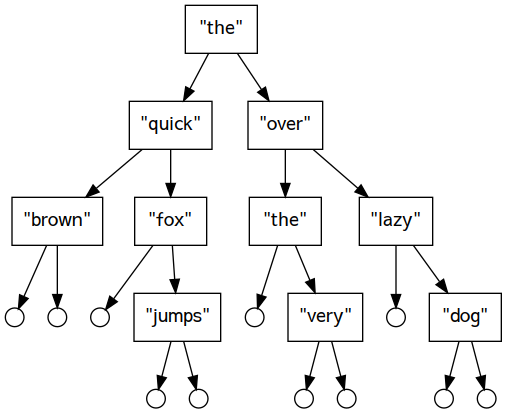The result of balancedFromList (words phrase)
phrase :: String
phrase = "the quick brown fox jumps over the very lazy dog"

# Generating figures

You don’t necessarily need to look at this section, but I’ll describe what it does in case you’re curious. The function graphviz takes a tree and converts it into a string in a language called GraphViz that can then be fed to a command-line tool to produce images. All the diagrams of trees on this page were produced from this Haskell program and GraphViz.

graphviz :: Show a => ITree a -> String
graphviz tree = surround $snd$ execRWS (travel tree) () 0
where
surround s = "digraph {\n" ++ s ++ "}\n"
quote a = "\"" ++ concatMap subst (show a) ++ "\""
subst '"' = "\\\""
subst c = [c]
increment :: MonadState Int m => (Int -> m a) -> m Int
increment f = do
i <- get
put (i+1)
void (f i)
return i
node i = "node" ++ show i
travel ILeaf = increment $\i -> tell$ node i ++ " [shape=circle, width=0.2, label=\"\"]\n"
travel (IBranch value left right) = increment $\i -> do j <- travel left k <- travel right tell$
node i ++ " [shape=rectangle, label=" ++ quote value ++ "]\n" ++
node i ++ " -> " ++ node j ++ "\n" ++
node i ++ " -> " ++ node k ++ "\n"
createGraph :: (String, String) -> IO ()
createGraph (name, dot) =
putStrLn ("Creating " <> filename) >>
withFile filename WriteMode (flip hPutStr dot)
where
filename = "a05" ++ name ++ ".dot"
main :: IO ()
main = mapM_ createGraph
[ ("t1", graphviz t1)
, ("t2", graphviz t2)
, ("t3", graphviz t3)
, ("t4", graphviz t4)
, ("t5", graphviz t5)
, ("t6", graphviz t6)
, ("t7", graphviz t7)
, ("t1sq", graphviz (fmap square t1))
, ("t1flip", graphviz (flipTree t1))
, ("buildints", graphviz (balancedFromList range))
, ("buildwords", graphviz (balancedFromList (words phrase)))
]

A compact string representation of trees:

compactShow :: Show a => ITree a -> String
compactShow ILeaf = "()"
compactShow (IBranch x ILeaf ILeaf) = show x
compactShow (IBranch x l r) =
concat [ "(", show x
, " ", compactShow l
, " ", compactShow r
, ")"
]

Used in some tests:

square :: Num a => a -> a
square x = x*x
twice :: Num a => a -> a
twice x = 2*x